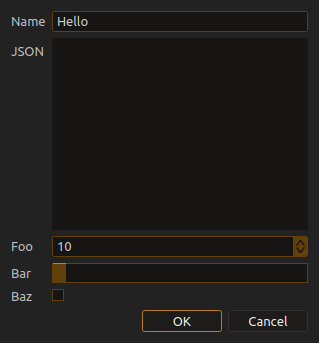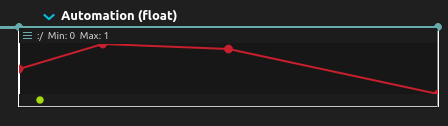# Utility functions

``````// Reads the entire content of a file

// Layouts a text so that it fits a rect of a given width by
// adding line breaks. Naive algorithm.
// Arguments are:
// - text to lay out
// - font to use
// - font size
// - maximum width in pixels
let text = Util.layoutTextLines("some long text", "Monospace", 24, 100);
``````

# Undo-redo and general helpers

``````// Find an object by name
let obj = Score.find("Interval.foobar");

// Get a reference to the object first selected in the score
let obj = Score.selectedObject();

// Get a reference to all the objects currently selected in the score
let objs = Score.selectedObjects();

// Get a reference to the current root document object
let doc = Score.document();

// Undo-redo:
Score.undo();
Score.redo();

// Undo-redo macros:
// this is so that commands that do multiple
// changes to the score, e.g. by adding multiple processes,
// only show up as one single action in the undo-redo panel.
// Every action that changes the score must be between these two calls.
Score.startMacro();
Score.endMacro();
``````

# UI

``````// This function allows to show a prompt to the user.
// The object passed in argument allows to configure the UI to show.
// They will be shown as a UI form.
// The return value will be the list of values that the user has set on every widget if the prompt was accepted, and 'undefined' otherwise.

const res = Score.prompt({
title: "Input JSON",
widgets: [
{ name:"Name", type: "lineedit", init: "Hello" },
{ name:"JSON", type: "textfield" },
{ name:"Foo", type: "spinbox", min: 0, max: 100, init: 10 },
{ name:"Bar", type: "slider", min: 0.5, max: 10.2, init: 1.0 },
{ name:"Baz", type: "checkbox", init: false }
]
});

// Res could look like:
// ["text", "long text", 30, 4.6, true]
``````# Transport

``````// Plays the score
Score.play();

// Plays a specific object
let an_interval = Score.find("my_interval");
Score.play(an_interval);

// Stops playback
Score.stop();
``````

# Functions operating on devices

``````// Converts a device tree to JSON.
// Useful for e.g. preset snapshotting, sending over the internet...
let json = Score.deviceToJson("deviceName");

// Create a WebSocket device from QML code.
// See the QML code examples in the library.
Score.createQMLWebSocketDevice("name", "QML Code");

// Create a Serial device from QML code.
// See the QML code examples in the library.
Score.createQMLSerialDevice("name", "/dev/ttyUSB1", "QML Code");

``````

# Functions operating on the score

``````// Create a new process in an interval.
// The process names are those in the library.
// Third argument is reserved for future use.
let new_process = Score.createProcess(an_interval, "Automation (float)", null);

// Change the name of an object
Score.setName(an_interval, "Foo");

// Create a new free-floating interval
// Arguments:
// - parent scenario
// - At which time the interval is created (e.g. "00:01:15.345" for 1 minute 15 seconds 345 millis)
// - How long it lasts (same format)
// - Which vertical position (between [0;1] (top;bottom))
let new_itv = Score.createBox(scenar, "00:00:10.000", "00:01:15.000", 0.2);

// Create a new interval after a state
let new_itv = Score.createIntervalAfter(state, duration, y);

// Get a port by name given a process
let port = Score.port(my_lfo, "Frequency");

// Get an inlet / outlet by index given a process.
// Here "port1" would be the "Offset" port of a LFO process
let port1 = Score.inlet(my_lfo, 2);
// Here "port2" would be the "Outlet" port of a LFO process
let port2 = Score.outlet(my_lfo, 0);

// Returns how many inlets / outlets there are in a given process
let count = Score.inlets(my_lfo);
let count = Score.outlets(my_lfo);

// Set the address of a port

// Set the value of a port (only relevant for ports with UI controls)
Score.setValue(port1, 0.34);
Score.setValue(portA, "foo");
Score.setValue(portB, true);
// etc...

// Get the type of a port as a string. Float, Int, String, etc.
let type = Score.valueType(port1);

// Get the min/max range of a port if it has one
let min = Score.min(port1);
let max = Score.max(port1);

// Get the list of values if the port is an enum / combo box / chooser ...
let vals = Score.enumValues(port1);

// Access the metadata object of an object. Allows to see the label, comments, etc
console.log(meta.name, meta.label, meta.comment)

// Access the start / end elements of an interval
let st = Score.startState(itv);
let st = Score.endState(itv);

let ev = Score.startEvent(itv);
let ev = Score.endEvent(itv);

let ts = Score.startSync(itv);
let ts = Score.endSync(itv);

// Remove an object from the score
Score.remove(itv);

// Create an automation on the given interval from an address
Score.automate(itv, "foo:/bar");

// Create an automation on the given interval from a port object
Score.automate(itv, port1);

``````

# Functions operating on curves

``````// Set a curve from a list of points:
// [ [ x1, y1 ], [ x2, y2 ], ... ]
let points = [
[0, 0.5],
[0.2, 1.],
[0.5, 0.9],
[1.0, 0.0],
];
Score.setCurvePoints(an_automation_process, points);
``````# Functions operating on step sequencer

``````// Sets the step on a Step Sequencer
Score.setSteps(a_step_sequencer_process, [0.1, 0, 1., 0.4, 0.5]);
``````